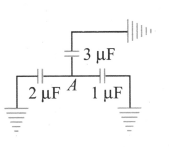Kirchhoff's rules for capacitors
Question

# In the given arrangement of capacitors, $6 \mu C,$ charge is added to point A. Find the charge on upper capacitorModerate
Solution

## $\frac{{q}_{1}}{1}=\frac{{q}_{2}}{2}=\frac{{q}_{3}}{3}=K$${q}_{1}+{q}_{2}+{q}_{3}=6$Solving, we get          K =1or ${q}_{3}=3 \mu C$

Get Instant Solutions